S k i l l
i n
A L G E B R A

20

# EXPONENTS II

Power of a fraction

Section 2

Power of a fraction"To raise a fraction to a power, raise the numerator
and denominator to that power."

 Example 1.For,  according to the meaning of the exponent and the rule for multiplying fractions:Example 2.   Apply the rules of exponents:Solution.   We must take the 4th power of everything.  But to take a power of a power -- multiply the exponents:Problem 1.   Apply the rules of exponents.

Do the problem yourself first!

 a)= x2y2 b)= 8x327 c)=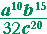d)=e)= x2 − 2x + 1x2 + 2x + 1

Perfect Square Trinomial

Dividing powers of the same base

In the next Lesson we will see the following rule for reducing a fraction:

 axay = xy

"Both the numerator and denominator may be divided
by a common factor."

Consider these examples:

 2 · 2 · 2 · 2 · 2     2 · 2 = 2 · 2 · 2
 ___2 · 2___2 · 2 · 2 · 2 · 2 = __1__2 · 2 · 2

If we write those with exponents, then22 = 23
 2225 = 1 23

In each case, we subtract the exponents.  But when the exponent in the denominator is larger, we write 1-over the difference.

 Example 3.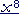x3 = x5x8 = 1 x5

Here is the rule: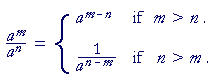Problem 2.   Simplify the following.  (Do not write a negative exponent.)

 a)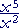= x3 b) x2x5 = 1 x3 c) xx5 = 1x4
 d) x2x = x e)= −x4 f)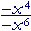= 1x2

Problem 3.   Simplify each of the following.  Then calculate each number.

 a)= 23 = 8 b) 2225 = 1 23 = 18 c) 225 = 124 = 1 16
 d) 222 = 2 e)= −24 = −16. See Lesson 13.
 f)= 122 = 14
 Example 4.   Simplify by reducing to lowest terms:Solution.   Consider each element in turn:Problem 4.   Simplify by reducing to lowest terms.  (Do not write negative exponents.

 a)= y35x3 b)= − 8a35b3
 c)= − 3z_5x4y3 d)= c316
 e) (x + 1)3 (x − 1)(x − 1)3 (x + 1) = (x + 1)2(x − 1)2

Negative exponents

We are now going to extend the meaning of an exponent to more than just a positive integer.  We will do that in such a way that the usual rules of exponents will hold.  That is, we will want the following rules to hold for any exponents:  positive, negative, 0 -- even fractions.

 aman = am + n Same Base (ab)n = anbn Power of a Product (am)n = amn Power of a Power

We begin by defining a number with a negative exponent.

 a−n = 1 an

It is the reciprocal of that number with a positive exponent.

an is the reciprocal of an.

 Example 5. 2−3 = 123 = 18

The base, 2, does not change.  The negative exponent becomes positive -- in the denominator.

Example 6.   Compare the following. That is, evaluate each one:

3−2    −3−2   (−3)−2    (−3)−3

 Answers. 3−2 = 132 = 19 .

Next,

−3−2 is the negative of 3−2. (See Lesson 13.)  The base is still 3.

 −3−2 = − 19 .

As for (−3)−2, the parentheses indicate that the base is −3:

 (−3)−2 = 1   (−3)2 = 19 .

Finally,

 (−3)−3 = 1   (−3)3 = − 1 27 .

A negative exponent, then, does not produce a negative number.  Only a negative base can do that. And then the exponent must be oddExample 7.   Simplify a2a5 .

Solution.   Since we have invented negative exponents, we can now subtract any exponents as follows:

 a2a5 = a2 − 5 = a−3

We now have the following rule for any exponents m, n: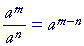In fact, it was because we wanted that rule to hold that we

 defined a− n as 1 an .

We want

 a2a5 = a−3

But

 a2a5 = 1 a3
 Therefore, we define  a−3 as 1 a3 .
 Example 8. a−1 = 1a

a−1 is now a symbol for the reciprocal, or multiplicative inverse, of any number a.  It appears in the following rule (Lesson 6):

a · a−1 = 1

Problem 5.   Evaluate the following.

 a)  ( 23 )−1 = 32 . 32 is the reciprocal of 23 .
 b)  ( 23 )−4 = 8116 . 8116 is the reciprocal of the 4th power of 23 .
 Problem 6.   Show:  am · b−n  = ambn .
 am · b−n  =  am · 1bn = ambn .

Example 9.   Use the rules of exponents to evaluate  (2−3 · 104)−2.

 Solution. (2−3 · 104)−2 = 26 · 10−8 Power of a power =Problem 6 = _64_      100,000,000

Problem 7.   Evaluate the following.

 a) 2−4 = 1 24 = 116 b) 5−2 = 1 52 = 125 c) 10−1 = 1 101 = 110
 d) (−2)−3 = 1   (−2)3 = 1  −8 = − 18
 e) (−2)−4 = 1   (−2)4 = 1 16 f) −2−4 = − 1 24 = − 1 16

g)  (½)−1 =  2.   2 is the reciprocal of ½.

Problem 8.   Use the rules of exponents to evaluate the following.

 a) 102 · 10−4 = 102 − 4 = 10−2 = 1/100.
 b) (2−3)2 = 2−6 = 1 26 = 1 64
 c) (3−2 · 24)−2 = 34 · 2−8 = 3428 = 81 256
 d) 2−2 · 2 = 2−2+1 = 2−1 = 12

Problem 9.   Rewrite without a denominator.

 a) x2x5 = x2−5 = x−3 b) yy6 = y1−6 = y−5
 c)= x−3y−4 d)= a−1b−6c−7
 e) 1x = x−1 f) 1x3 = x−3
 g) (x + 1)    x = (x + 1)x−1 h) (x + 2)2(x + 2)6 = (x + 2)−4

Example 10.     Rewrite without a denominator, and evaluate:Answer.   The rule for subtracting exponents ---- holds even when an exponent is negative.Therefore,= 10−3 + 5 − 2 + 4 = 104 = 10,000.

Exponent 2 goes into the numerator as −2;  exponent −4 goes there as +4.

Problem 10.    Rewrite without a denominator and evaluate.

 a) 222−3 = 22 + 3  = 25 = 32 b) 10210−2 = 102 + 2  = 104 = 10,000
 c)= 102 − 5 − 4 + 6  = 10−1 = 1 10
 d)= 25 − 6 + 9 − 7  = 21 = 2

Problem 11.   Factors may be shifted between the numerator and denominator [How?]

By changing the sign of the exponent.

 Example 11.   Rewrite without a denominator:Answer.The exponent 3 goes into the numerator as −3;  the exponent −4 goes there as +4.

Problem 12.    Rewrite with positive exponents only.

 a) x y−2 = xy2 b)=c)=d)=e)=Problem 13.    Apply the rules of exponents, then rewrite with positve exponents.

 a)==b)==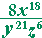Section 2: Exponent 0Next Lesson:  Algebraic fractions

Please make a donation to keep TheMathPage online.
Even \$1 will help.